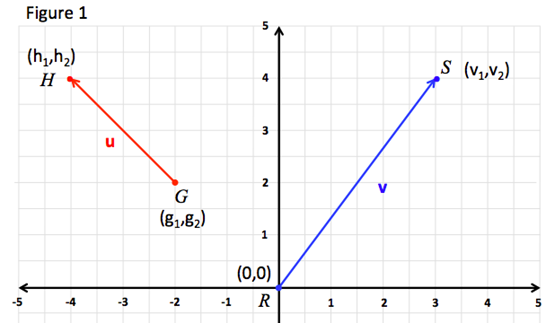# Component Form and Magnitude

A vector is a quantity with both magnitude and direction. To differentiate vectors from variables, constants and imaginary numbers vectors are denoted by a lowercase boldface variable, v, or a variable with a harpoon arrow above it, $\stackrel{⇀}{v}$.

In this discussion vectors will be denoted by lowercase boldface variables. Examples of vector quantities are velocity, acceleration and force.

Figure 1 shows two vectors v and u as directed line segments.The vector v is represented by the directed line segment $\stackrel{⇀}{RS}$ and has an initial point at R and a terminal point at S.

Vectors are often represented in component form. Vector v in Figure 1 has an initial point at the origin (0,0) and is said to be in standard position. A vector in standard position can be represented by the coordinates of its terminal point. Thus vin component form = $〈{v}_{1},{v}_{2}〉$.

Notice that the brackets surrounding the vector components v1 and v2 are pointed not round like parentheses.

The magnitude of v or $\stackrel{⇀}{RS}$ is represented by $\parallel \stackrel{⇀}{RS}\parallel$ or $\parallel v\parallel$ and is calculated using the Distance Formula, $\parallel v=\sqrt{{v}_{1}^{2}+{v}_{2}^{2}}\parallel$.

MAGNITUDE OF A VECTOR:

$\parallel v=\sqrt{{v}_{1}^{2}+{v}_{2}^{2}}\parallel$

Vector u represented by $\stackrel{⇀}{GH}$ in Figure 1 is not in standard position as its initial point is not the origin (0,0). The component form and magnitude of vector u can be calculated as follows:

COMPONENT FORM OF DIRECTED LINE SEGMENT:

Initial point:         G (g1, g2)
Terminal Point:     H (h1, h2)

MAGNITUDE OF DIRECTED LINE SEGMENT:

Initial point:         G (g1, g2)
Terminal Point:     H (h1, h2)

$\stackrel{⇀}{\parallel GH\parallel }=\sqrt{{\left({h}_{1}-{g}_{1}\right)}^{2}+{\left({h}_{2}-{g}_{2}\right)}^{2}}=\sqrt{{u}_{1}^{2}+{u}_{2}^{2}}$

Let's look at a couple examples.

Example 1:      Find the component form and magnitude of vector u in Figure 1.
 Step 1: Identify the initial and terminal coordinates of the vector. Initial Point G: (-2, 2) Terminal Point H: (-4, 4) Step 2: Calculate the components of the vector. Subtract the x-component of the terminal point from the x-component of the initial point for your x-component of the vector. Do the same for the y-components. Step 3: Calculate the magnitude of the vector. ||u|| = $\sqrt{{u}_{1}^{2}+{u}_{2}^{2}}$ ||u|| = $\sqrt{{\left(-2\right)}^{2}+{\left(2\right)}^{2}}$ $\sqrt{4+4}$ $\sqrt{8}$
Example 2:      Find the component form and magnitude of vector v in standard position with terminal point (8, -2).
 Step 1: Identify the initial and terminal coordinates of the vector. Because vector v is in standard position it's initial point is (0,0) Initial Point: (0, 0) Terminal Point: (8, -2) Step 2: Calculate the components of the vector. Subtract the x-component of the terminal point from the x-component of the initial point for your x-component of the vector. Do the same for the y-components. In this case the vector is in standard form therefore the components of the vector are the same as the components of the terminal point. $〈8,-2〉=v$ Step 3: Calculate the magnitude of the vector. ||v|| = $\sqrt{{v}_{1}^{2}+{v}_{2}^{2}}$ ||v|| = $\sqrt{{\left(8\right)}^{2}+{\left(-2\right)}^{2}}$ $\sqrt{64+4}$ $\sqrt{68}$ $2\sqrt{17}$

 Related Links: Math algebra Scalar Multiplication and Vector Addition Unit Vectors Pre Calculus

To link to this Component Form and Magnitude page, copy the following code to your site: